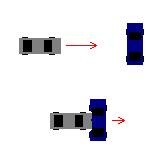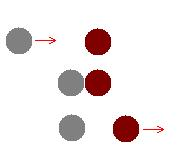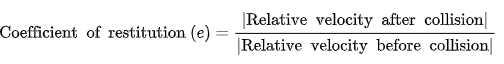## A Car Crash

Consider a car runs a red light. When the vehicle collides with another car, is energy conserved? What about momentum?Hint
In this scenario, an inelastic collision occurred, in that two objects do not bounce away from each other upon impact.
Hint 2
Are there any losses due to conversions into sound, heat, deformation etc.?
In this scenario, an inelastic collision occurred, in that two objects do not bounce away from each other upon impact. Momentum is conserved, because the total momentum of both objects before and after the collision is the same. However, kinetic energy is not conserved. Some of the kinetic energy is converted into sound, heat, and deformation of the objects. If you calculated the momentum of the cars before the collision and added it together, it would be equal to the momentum after the collision when the two cars are stuck together. However, if you calculated the kinetic energy before and after the collision, you would find some of it had been converted to other forms of energy.If an elastic collision were to occur (like two balls bouncing apart after impact), both momentum and kinetic energy are conserved. Almost no energy is lost to sound, heat, or deformation. The first rubber ball deforms, but then quickly bounces back to its former shape, and transfers almost all the kinetic energy to the second ball. Energy is only conserved if the restitution coefficient equals 1.Momentum is conserved, while kinetic energy is not.